# A disabled automobile is pulled by means of two ropes as shown. Knowing that the tension in rope AB is 3 kN, determine by trigonometry the tension in rope AC and the value of α so that the resultant force exerted at A is a 4.8-kN force directed along the axis of the automobile.

Question-AnswerCategory: Engineering MechanicsA disabled automobile is pulled by means of two ropes as shown. Knowing that the tension in rope AB is 3 kN, determine by trigonometry the tension in rope AC and the value of α so that the resultant force exerted at A is a 4.8-kN force directed along the axis of the automobile.

### A disabled automobile is pulled by means of two ropes as shown. Knowing that the tension in rope AB is 3 kN, determine by trigonometry the tension in rope AC and the value of α so that the resultant force exerted at A is a 4.8-kN force directed along the axis of the automobile.Fig. P2.9Step: 1

Draw the force triangle.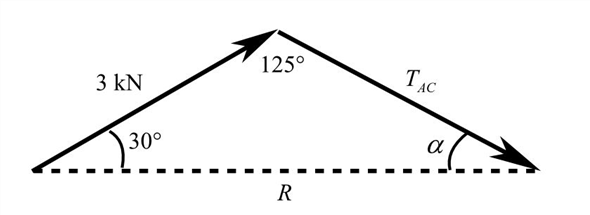Step: 2

Use cosines law to find the tension in rope AC.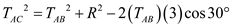Here, tension in the cable AB is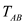and the resultant force is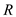.
Substitute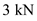forandfor.Therefore, tension in the rope AC is.

Step: 3

Use law of sine to find the direction of the tension in rope AC.Substitute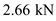for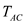.Direction of the tension in rope AC is.